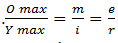## Mechanics of Materials Question Paper of 3rd Semester AE Download Previous Years Question Paper 6

•Tuesday, August 30, 2016
••,
•No comments

Roll No. ......................
Total No. of Questions : 09]
B. Tech. (Sem. – 3rd)
MECHANICS OF MATERIALS
SUBJECT CODE : BTAE-301
Paper ID : [A1112]
Time : 03 Hours
Instruction to Candidates:
1. SECTION-A is COMPULSORY consisting of TEN questions carrying
TWO marks each.
2. SECTION-B contains FIVE questions carrying FIVE marks each and
students has to attempt any FOUR questions.
3. SECTION-C contains THREE questions carrying TEN marks each and
students has to attempt any TWO questions.

SECTION-A
1. Write briefly :
(a) What do you mean by Poisson’s Ratio?
(b) Define Hoop Stress and Longitudinal Stress.
(c) Write down the formula of power transmitted by hollow shaft subjected
to pure torsion.
(d) What do you understand by Principle Stress?
(e) Define Slenderness Ratio.
(f) What do you mean by Temperature Stress?
(g) What do you understand by Fletched beam?
(h) State Maximum shear stress theory of failure.
(i) Define shear stress. Write the formula of maximum shear stress in a
circular section beam.
(j) Calculate the maximum bending moment in a simply supported beam
of length 5m and carrying a concentrated load of intensity 10 N at the centre.
SECTION-B

2. Draw the shear force diagram and bending moment diagram for a simply
supported beam having uniformly distributed load over its whole span.
3. A thin cylinder 2m long. 0.5 m in diameter and made out of 10 mm thick
steel plate is subjected to an internal pressure of 2MPa. Calculate the change in diameter and change in length under pressure. Take Young’s Modulus as 200GPa and Poisson’s Ratio 0.25.
4. Show that the elongation of conical bar of length ‘L’ and base diameter
‘D’ under its own weight is given by gL2/6E. Where. E is Young’s
Modulus, density of material is  and g is acceleration due to gravity.
5. A hollow circular shaft is required to transmit 500 kW of power at
100 rpm. Determine, the diameters of shaft if the inside diameter is
0.8 times the outside diameter. Take allowable shear stress as 75 MPa.
6. Derive an expression for the crippling load for a both end fixed column,
according to Euler’s theory. State the assumptions made.

SECTION-C

7. A solid circular shaft is required to carry a torque of 40 kNm and a
bending moment of 30 kNm. If yield stress in a simple tensile test on the
material of the shaft was found to be 260 N/mm2. Find the minimum
required diameter of the shaft according to
(a) shear stress theory
(b) von-Misses Theory
Take a factor of Safety = 2.5.
8. A beam simply supported over a span of 10 m carries concentrated loads
of 40 kN. 20 kN and 60 kN at 2m. 5m and 9m from the left end
support. Determine the position and amount of maximum deflection in the
beam by using Macaulay’s Method. Take I = 695 × 106 mm4 and
E = 200 GPa
9. What do you mean by pure Bending? Derive the Bending equation for a

straight beam subjected to pure bending i.e. .Also write the assumptions made in the deriving the bending equation.